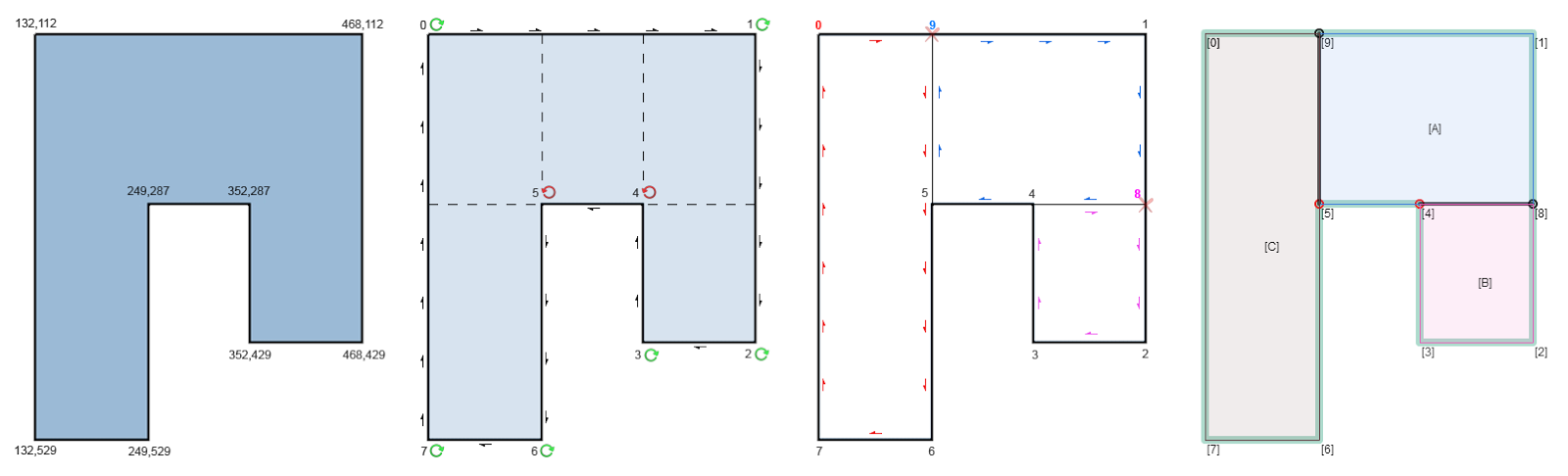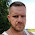## 2.7.14

### Orthogonal Polygon Partitioning

I got a request to implement an algorithm which divides a polygon into rectangles. The polygon only consists of right angles and no holes. The least amount of rectangular shaped partitions is desired. And oh boy was it fun!

The source-code for Haxe, PHP and JS as well as a Flash-AS3 demo can be found on my Git-Hub.input, divide, extract, result
1. traverse the polygon clockwise and find vertices with counterclockwise rotation
2. split the graph from those vertices to any direction
3. pick a vertex and traverse the adjusted graph clockwise to build your rectangles

My first approach was very different from the final solution and did not use a graph-traversal but recursively partitioned its bounding into smaller rectangles.first approach
I started with a bounding rectangle tightly surrounding the given polygon and then split it on each vertex inside the bounding on the x and y axis. I created for each vertex 4 areas, discarded the empty ones and later determined whether or not the resulting partitions are inside of the original polygon.

It might have been my implementation but on more complex polygons this approach started to fall apart and missed a lot of partitions or created more than necessary. The algorithm I used to exclude areas outside of the polygon gave me an idea how to solve this problem more elegant.

The first goal is to find all vertices building an obtuse angle which are the very vertices preventing the polygon from closing. The second step is to add a closed walk to the graph using those vertices and the last step is to find all cycles and build the required partitions.

Splitting Counter-Clockwise:
There is a technique in 3D rendering software called backface culling used to remove hidden surfaces of polygons facing away from the camera. I recently implemented it on my own and there is a little math involved but the idea is to define a surface of at least 3 vertices and find its normal. The important and useful property of the calculation involved is that depending on the order of those vertices the direction of the normal changes. [illustration] In the first example its Vertex 4 and 5.
```private function isClockwise( a:Vector2, b:Vector2, c:Vector2 ):Bool
{
var p1:Vector3 = new Vector3( a.x, a.y, 0 );
var p2:Vector3 = new Vector3( b.x, b.y, 0 );
var p3:Vector3 = new Vector3( c.x, c.y, 0 );

var sub1:Vector3 = Vector3.subtract( p2, p1, new Vector3() );
var sub2:Vector3 = Vector3.subtract( p3, p1, new Vector3() );

return Vector3.cross( sub1, sub2 ).z > 0;
}```
I traverse the previously built polygon graph and call the function above for each vertex (b) with its 2 adjacent neighbor vertices (a previous, c next). For each vertex returning false I proceed with the second step: Splitting the graph.

The split is done calculating a ray normal to the edge defined by the counter-clockwise vertex and its previous node and checking each edge for intersection. To minimize the checks required I keep 2 sorted lists for the edges. Vertical edges sorted on the x-axis from left to right and horizontal edges on the y-axis from top to bottom. Depending on the direction of the ray I compare the closest edges for intersection first.

In the example I cast a ray starting from the vertex 4 to the right. I look up where the edge 3,4 would be in my sorted edge-list and only consider all edges to the right of it. Once I find an edge where the rays y-axis lies within I insert a new vertex and horizontal edge, adjust the graph connections and proceed with the next counter-clockwise vertex.

Although it is actually a simple task there is quite some code involved and I unfortunately made the mistake to optimize too early. The responsible EdgeContainer-Class does not work with a custom datatype for edges and the duplicated code for each axis (vertical and horizontal) further obfuscate the code. Sorting the edges and checking them in the right direction seemed to be necessary though to avoid splitting wrong edges.

Extracting Partitions:
At this point the polygon is not a single cycle graph anymore but contains several closed walks. The tricky part now is to get the rectangular areas defined by those. The idea is to pick a random vertex and start traversing the graph in the most clockwise way possible to find the those closed walks. Those vertices can then be used to generate a rectangle. But how do I choose which node to pick while traversing when there are now multiple paths at some vertices like in the example 4,5,8 and 9?

A smart and clean way would be to utilize the trusty isClockwise method. You select at least 3 nodes and for each iteration keep your previous 2 vertices and try with a third. If those 3 vertices are counter-clockwise you pick another node until you've found a clockwise one.
```var rotation:Array<Vector2> = new Array<Vector2>();
rotation = new Vector2(  1,  0 );    // right
rotation = new Vector2(  0,  1 );    // down
rotation = new Vector2( -1,  0 );    // left
rotation = new Vector2(  0, -1 );    // up```
A naive and painful way would be to hardcode the rotation and pick order only to realize that this would run into even worse try-and-error cases as some vertices simply don't have a node to the right or bottom.

Which is exactly what I did.complicated example
Independent of the way you actually traverse a small closed walk to find a partition is the problem of avoiding duplicated areas. If you iterate over each vertex and start your area extraction it is very likely that you get to the same result you previously did. In the complicated example above I would get the same partition H for the starting vertices 4 or 5.

I guess you could only select vertices that are, lets say, top-right corners and go from there. I didn't investigate this problem further as its a performance impact I can live with (besides having tighter bottlenecks) and simply discarded duplicates.

Maybe I clean it up though, only time will tell ...

1.Great job!
Do you have an AS3 version of code and lib? Or do you mind if I port it?

2.Crashes in that case:
740 917 740 747 540 747 540 637 390 637 390 527 260 527 260 427 130 427 130 177 710 177 710 87 950 87 950 257 1090 257 1090 387 1220 387 1220 567 1340 567 1340 767 1080 767 1080 917

Screen:
http://joxi.ru/752a1L4i4PjR20

1.hello, thank you. I updated the version to include AS3 source code and fixed the bug you pointed out. feel free to port, fork and use it as you wish :)